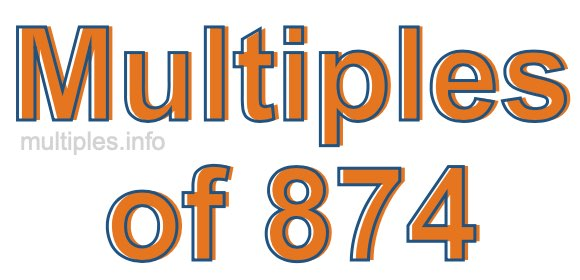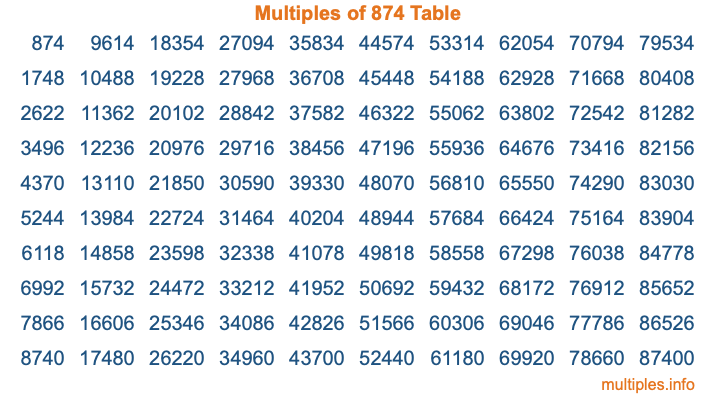Multiples of 874Welcome to the Multiples of 874 page. Here we will first teach you everything you will ever need to know about the multiples of 874, and then give you a study guide summary of everything we taught you to make sure you remember it all. Use this page to look up facts and learn information about the multiples of 874. This page will make you a multiples of eight hundred seventy-four expert!

Definition of Multiples of 874
Multiples of 874 are all the numbers that when divided by 874 equal an integer. Each of the multiples of 874 are called a multiple. A multiple of 874 is created by multiplying 874 by an integer.

Therefore, to create a list of multiples of 874, you start with 1 multiplied by 874, then 2 multiplied by 874, then 3 multiplied by 874, and so on for as long as you want. Thus, the list of the first five multiples of 874 is 874, 1748, 2622, 3496, and 4370. To see a larger list of multiples of 874, see the printable image of Multiples of 874 further down on this page. We also have a category where you can choose any nth multiple of 874.

Multiples of 874 Checker
The Multiples of 874 Checker below checks to see if any number of your choice is a multiple of 874. In other words, it checks to see if there is any number (integer) that when multiplied by 874 will equal your number. To do that, we divide your number by 874. If the the quotient is an integer, then your number is a multiple of 874.

Is  a multiple of 874?

Least Common Multiple of 874 and ...
A Least Common Multiple (LCM) is the lowest multiple that two or more numbers have in common. This is also called the smallest common multiple or lowest common multiple and is useful to know when you are adding our subtracting fractions. Enter one or more numbers below (874 is already entered) to find the LCM.

Check out our LCM Calculator if you need more details about the Least Common Multiple or if you need the LCM for different numbers for adding and subtraction fractions.

nth Multiple of 874
As we stated above, 874 is the first multiple of 874, 1748 is the second multiple of 874, 2622 is the third multiple of 874, and so on. Enter a number below to find the nth multiple of 874.

th multiple of 874

Multiples of 874 vs Factors of 874
874 is a multiple of 874 and a factor of 874, but that is where the similarities end. All postive multiples of 874 are 874 or greater than 874. All positive factors of 874 are 874 or less than 874.

Below is the beginning list of multiples of 874 and the factors of 874 so you can compare:

Multiples of 874: 874, 1748, 2622, 3496, 4370, etc.

Factors of 874: 1, 2, 19, 23, 38, 46, 437, 874

As you can see, the multiples of 874 are all the numbers that you can divide by 874 to get a whole number. The factors of 874, on the other hand, are all the whole numbers that you can multiply by another whole number to get 874.

It's also interesting to note that if a number (x) is a factor of 874, then 874 will also be a multiple of that number (x).

Multiples of 874 vs Divisors of 874
The divisors of 874 are all the integers that 874 can be divided by evenly. Below is a list of the divisors of 874.

Divisors of 874: 1, 2, 19, 23, 38, 46, 437, 874

The interesting thing to note here is that if you take any multiple of 874 and divide it by a divisor of 874, you will see that the quotient is an integer.

Multiples of 874 Table
Below is an image of the first 100 multiples of 874 in a table. The table is in chronological order, column by column. The first column has the first ten multiples of 874, the second column has the next ten multiples of 874, and so on.The Multiples of 874 Table is also referred to as the 874 Times Table or Times Table of 874. You are welcome to print out our table for your studies.

Negative Multiples of 874
Although not often discussed or needed in math, it is worth mentioning that you can make a list of negative multiples of 874 by multiplying 874 by -1, then by -2, then by -3, and so on, to get the following list of negative multiples of 874:

-874, -1748, -2622, -3496, -4370, etc.

Multiples of 874 Summary
Below is a summary of important Multiples of 874 facts that we have discussed on this page. To retain the knowledge on this page, we recommend that you read through the summary and explain to yourself or a study partner why they hold true.

There are an infinite number of multiples of 874.

A multiple of 874 divided by 874 will equal a whole number.

874 divided by a factor of 874 equals a divisor of 874.

The nth multiple of 874 is n times 874.

The largest factor of 874 is equal to the first positive multiple of 874.

874 is a multiple of every factor of 874.

874 is a multiple of 874.

A multiple of 874 divided by a divisor of 874 equals an integer.

874 divided by a divisor of 874 equals a factor of 874.

Any integer times 874 will equal a multiple of 874.

Multiples of a Number
Here you can get the multiples of another number, all with the same attention to detail as we did for multiples of 874 on this page.

Multiples of
Multiples of 875
Did you find our page about multiples of eight hundred seventy-four educational? Do you want more knowledge? Check out the multiples of the next number on our list!